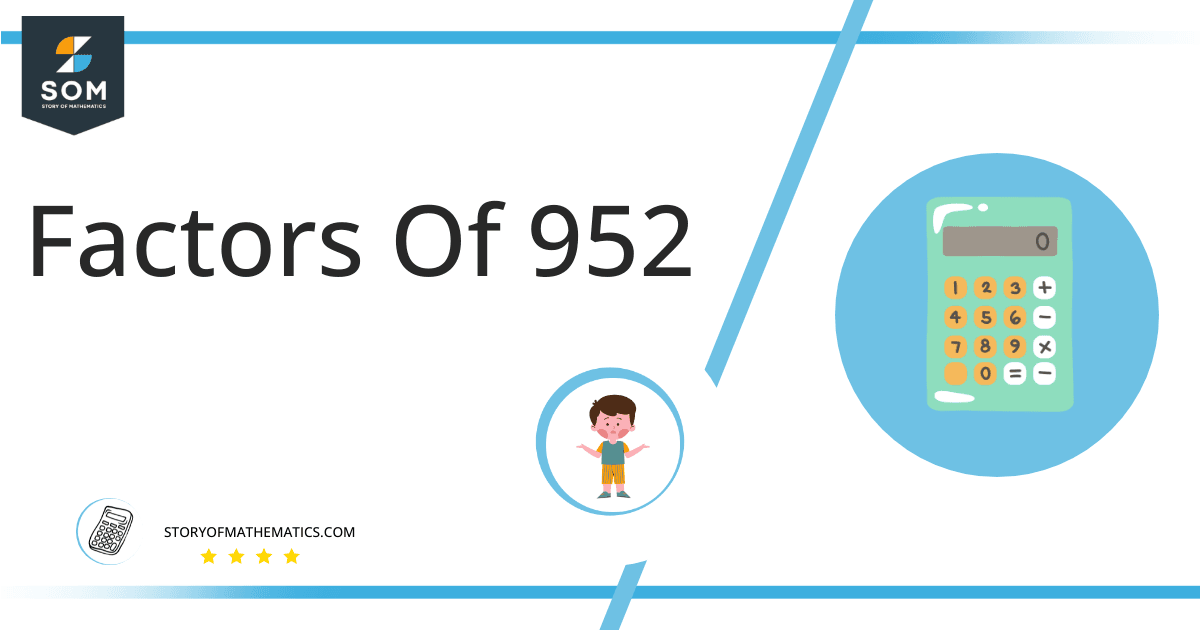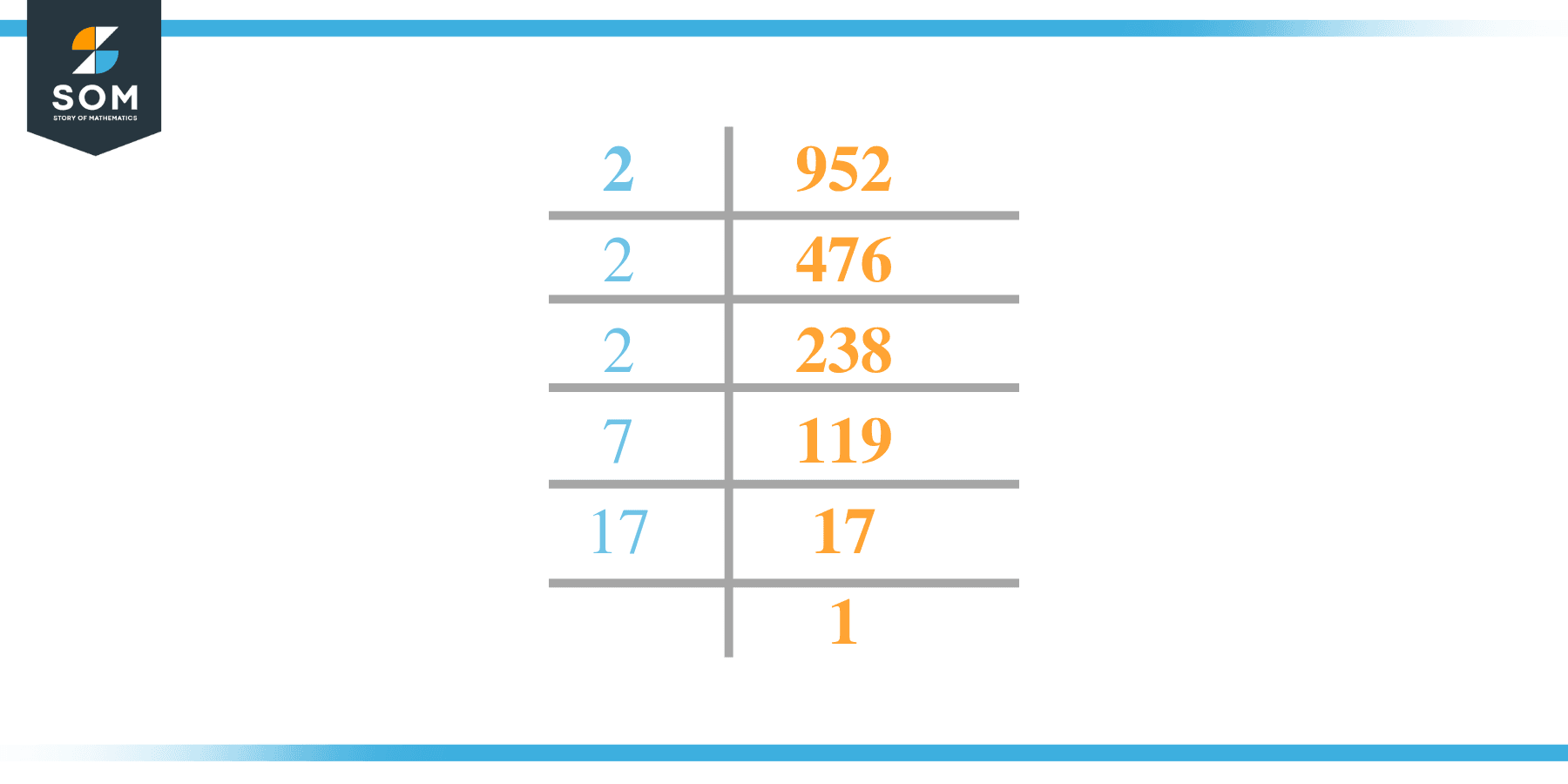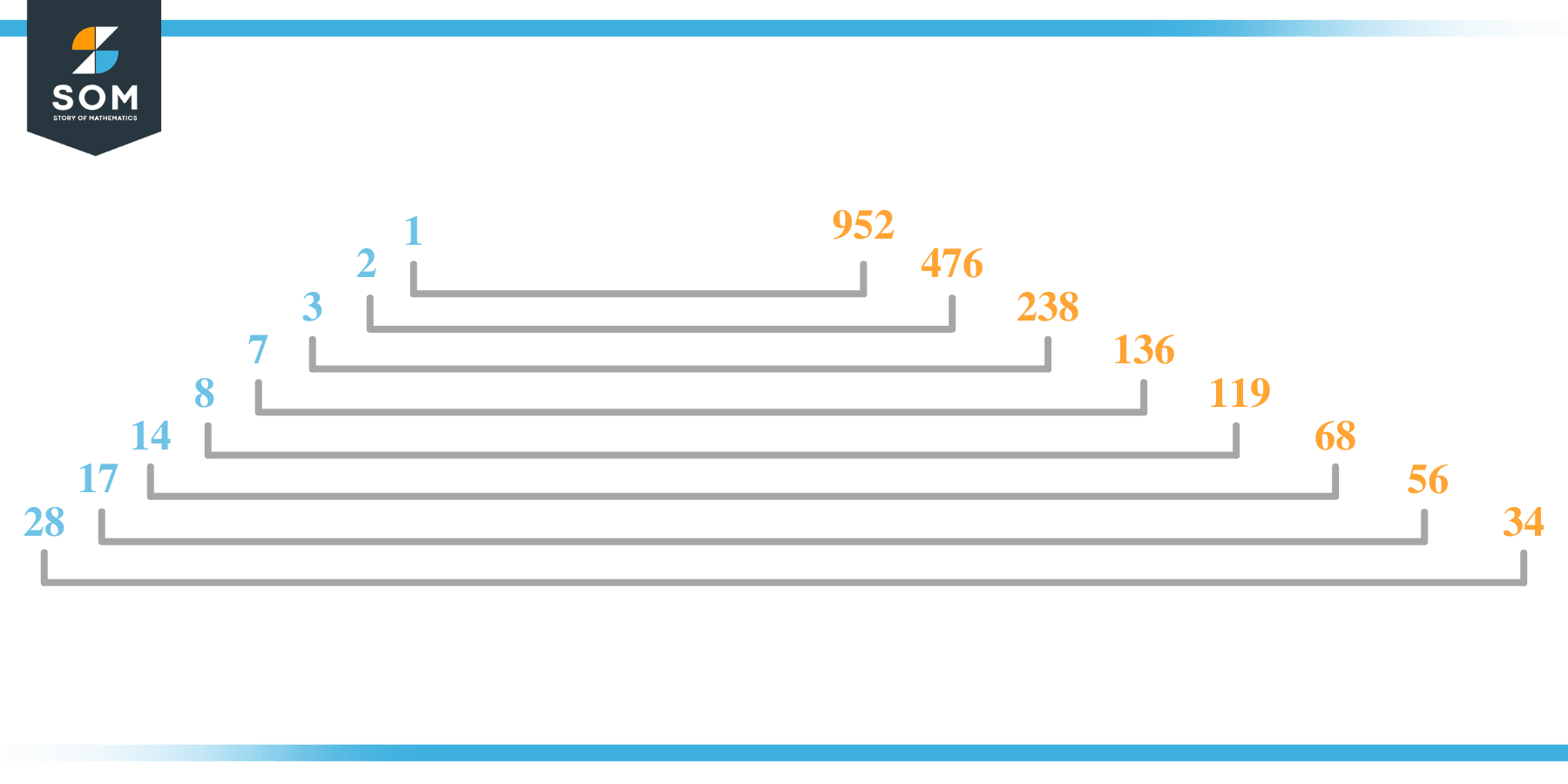# Factors of 952: Prime Factorization, Methods, and Examples

The factors of 952 are the numbers that are divisible by 952. The number 952 is an even number. It has more than two factors which is why 952 is called a composite number.Let us explore more about the factors of 952.

### Factors of 952

Here are the factors of number 952.

Factors of 952: 1, 2, 4, 7, 8, 14, 17, 28, 34, 56, 68, 119, 136, 238, 476, and 952

### Negative Factors of 952

The negative factors of 952 are similar to their positive aspects, just with a negative sign.

Negative Factors of 952: –1, -2, -4, -7, -8, -14, -17, -28, -34, -56, -68, -119, -136, -238, -476, and -952

### Prime Factorization of 952

The prime factorization of 952 is the way of expressing its prime factors in the product form.

Prime Factorization: 2 x 2 x 2 x 7 x 17

In this article, we will learn about the factors of 952 and how to find them using various techniques such as upside-down division, prime factorization, and factor tree.

## What Are the Factors of 952?

The factors of 952 are 1, 2, 4, 7, 8, 14, 17, 28, 34, 56, 68, 119, 136, 238, 476, and 952. These numbers are the factors as they do not leave any remainder when divided by 952.

The factors of 952 are classified as prime numbers and composite numbers. The prime factors of the number 952 can be determined using the prime factorization technique.

## How To Find the Factors of 952?

You can find the factors of 952 by using the rules of divisibility. The divisibility rule states that any number, when divided by any other natural number, is said to be divisible by the number if the quotient is the whole number and the resulting remainder is zero.

To find the factors of 952, create a list containing the numbers that are exactly divisible by 952 with zero remainders. One important thing to note is that 1 and 952 are 952’s factors, as every natural number has 1 and the number itself as its factor.

1 is also called the universal factor of every number. The factors of 952 are determined as follows:

$\dfrac{952}{1} = 952$

$\dfrac{952}{2} = 476$

$\dfrac{952}{4} = 238$

$\dfrac{952}{7} = 136$

$\dfrac{952}{8} = 119$

$\dfrac{952}{14} = 68$

$\dfrac{952}{17} = 56$

$\dfrac{952}{28} = 34$

Therefore, 1, 2, 4, 7, 8, 14, 17, 28, 34, 56, 68, 119, 136, 238, 476, and 952 are the factors of 952.

### Total Number of Factors of 952

For 952, there are sixteen positive factors and sixteen negative ones. So in total, there are thirty-two factors of 952.

To find the total number of factors of the given number, follow the procedure mentioned below:

1. Find the factorization/prime factorization of the given number.
2. Demonstrate the prime factorization of the number in the form of exponent form.
3. Add 1 to each of the exponents of the prime factor.
4. Now, multiply the resulting exponents together. This obtained product is equivalent to the total number of factors of the given number.

By following this procedure, the total number of factors of X is given as:

The factorization of 952 is 1 x 2$^3$ x 7 x 17.

The exponent of 1, 7, and 17 is 1. The exponent of 2 is 3.

Adding 1 to each and multiplying them together results in 32.

Therefore, the total number of factors of 952 is 32. Sixteen are positive, and sixteen factors are negative.

### Important Notes

Here are some essential points that must be considered while finding the factors of any given number:

• The factor of any given number must be a whole number.
• The factors of the number cannot be in the form of decimals or fractions.
• Factors can be positive as well as negative.
• Negative factors are the additive inverse of the positive factors of a given number.
• The factor of a number cannot be greater than that number.
• Every even number has 2 as its prime factor, the smallest prime factor.

## Factors of 952 by Prime FactorizationThe number 952 is a composite. Prime factorization is a valuable technique for finding the number’s prime factors and expressing the number as the product of its prime factors.

Before finding the factors of 952 using prime factorization, let us find out what prime factors are. Prime factors are the factors of any given number that are only divisible by 1 and themselves.

To start the prime factorization of 952, start dividing by its most minor prime factor. First, determine that the given number is either even or odd. If it is an even number, then 2 will be the smallest prime factor.

Continue splitting the quotient obtained until 1 is received as the quotient. The prime factorization of 952 can be expressed as:

952 = 2$^3$ x 7 x 17

## Factors of 952 in PairsThe factor pairs are the duplet of numbers that, when multiplied together, result in the factorized number. Factor pairs can be more than one depending on the total number of factors given.

For 952, the factor pairs can be found as:

1 x 952 = 952

2 x 476 = 952

4 x 238 = 952

7 x 136 = 952

8 x 119 = 952

14 x 68 = 952

17 x 56 = 952

28 x 34 = 952

The possible factor pairs of 952 are given as (1, 952), (2, 476), (4, 238), (7, 136), (8, 119), (14, 68), (17, 56), and (28, 34).

All these numbers in pairs, when multiplied, give 952 as the product.

The negative factor pairs of 952 are given as:

-1 x -952 = 952

-2 x -476 = 952

-4 x -238 = 952

-7 x -136 = 952

-8 x -119 = 952

-14 x -68 = 952

-17 x -56 = 952

-28 x -34 = 952

It is important to note that in negative factor pairs, the minus sign has been multiplied by the minus sign, due to which the resulting product is the original positive number. Therefore, 1, -2, -4, -7, -8, -14, -17, -28, -34, -56, -68, -119, -136, -238, -476, and -952 are called negative factors of 952.

The list of all the factors of 952, including positive as well as negative numbers, is given below.

Factor list of 952: 1, -2, -4, -7, -8, -14, -17, -28, -34, -56, -68, -119, -136, -238, -476, and -952

## Factors of 952 Solved Examples

To better understand the concept of factors, let’s solve some examples.

### Example 1

How many factors of 952 are there?

### Solution

The total number of Factors of 952 is sixteen.

Factors of 952 are 1, 2, 4, 7, 8, 14, 17, 28, 34, 56, 68, 119, 136, 238, 476, and 952.

### Example 2

Find the factors of 952 using prime factorization.

### Solution

The prime factorization of 952 is given as:

952 $\div$ 2 = 476

476 $\div$ 2 = 238

238 $\div$ 2 = 119

119 $\div$ 7 = 17

17 $\div$ 17 = 1

So the prime factorization of 952 can be written as:

2 x 2 x 2 x 7 x 17 = 952